# 「力扣」第 1081 题：不同字符的最小子序列

## 「力扣」第 1081 题：不同字符的最小子序列

1 <= text.length <= 1000
text 由小写英文字母组成

## 贪心算法 + 栈、位掩码（Python 代码、Java 代码）

### 理解题意

##### 1、字典序

aabc < aacb < abac

##### 2、子序列

（1）并不要求是连续子序列，例如 aabc 的子序列之一可以是 ac
（2）子序列中各个字符的相对顺序应该与原字符串一致，例如 ca 就不是 aabc 的子序列；
（3）子序列包含 text 中所有不同字符一次，这里注意关键词 “所有” 和 “一次”，所以要求我们找出的子序列包含的字符应该是 “不重不漏” 的。

### 思路分析

（温馨提示：下面的幻灯片中，有几页上有较多的文字，可能需要您停留一下，可以点击右下角的后退 “|◀” 或者前进 “▶|” 按钮控制幻灯片的播放。）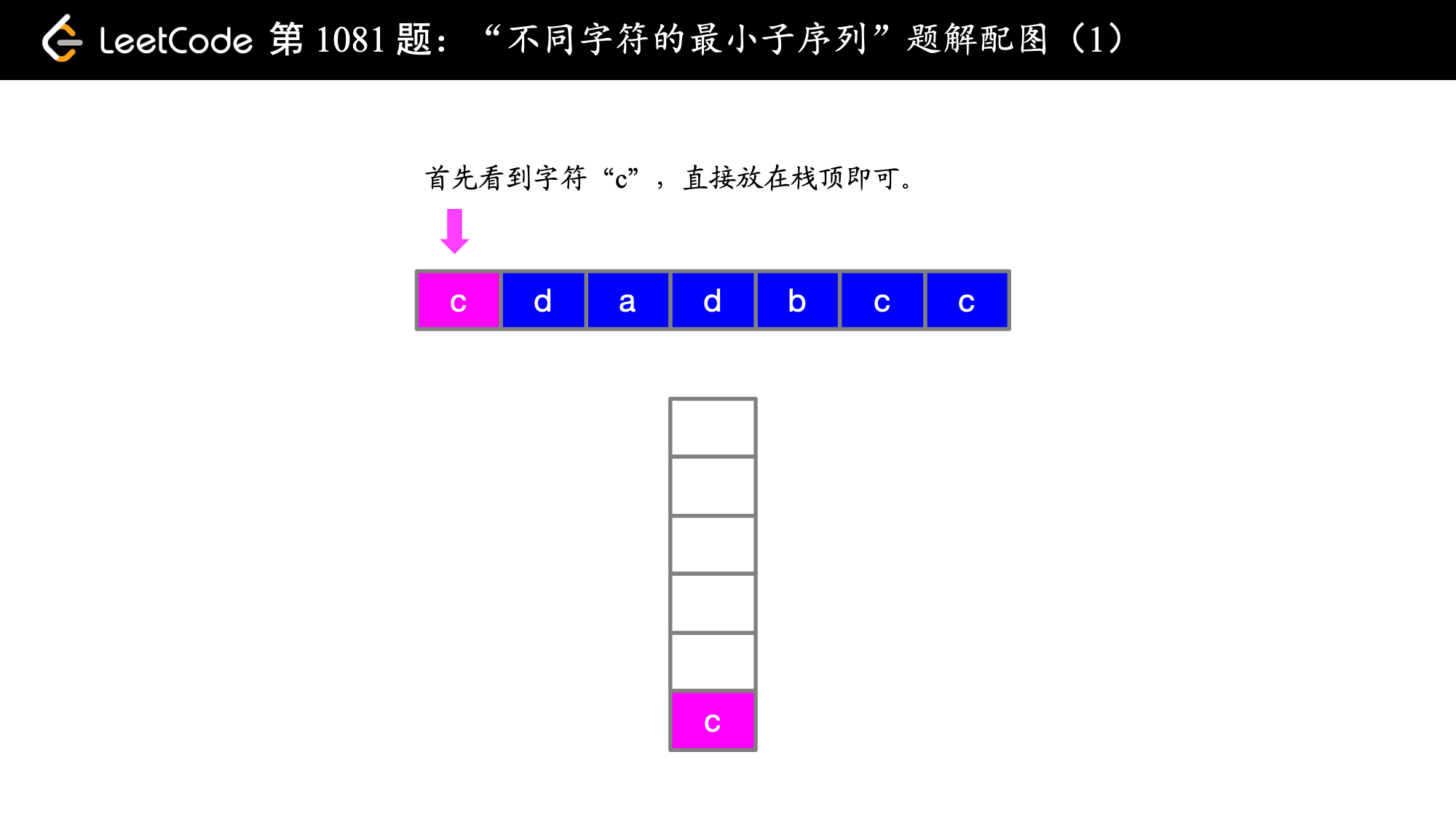),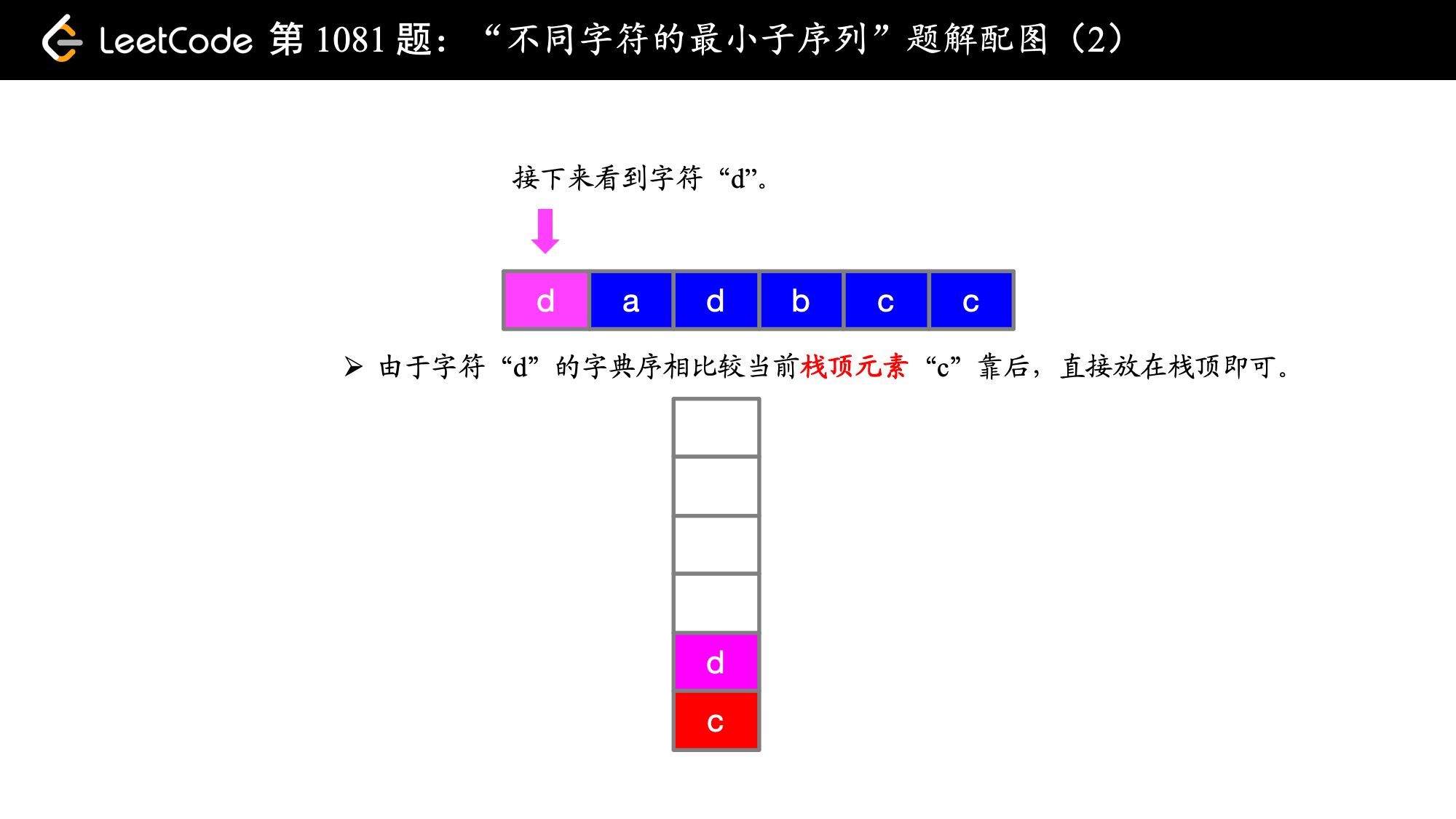),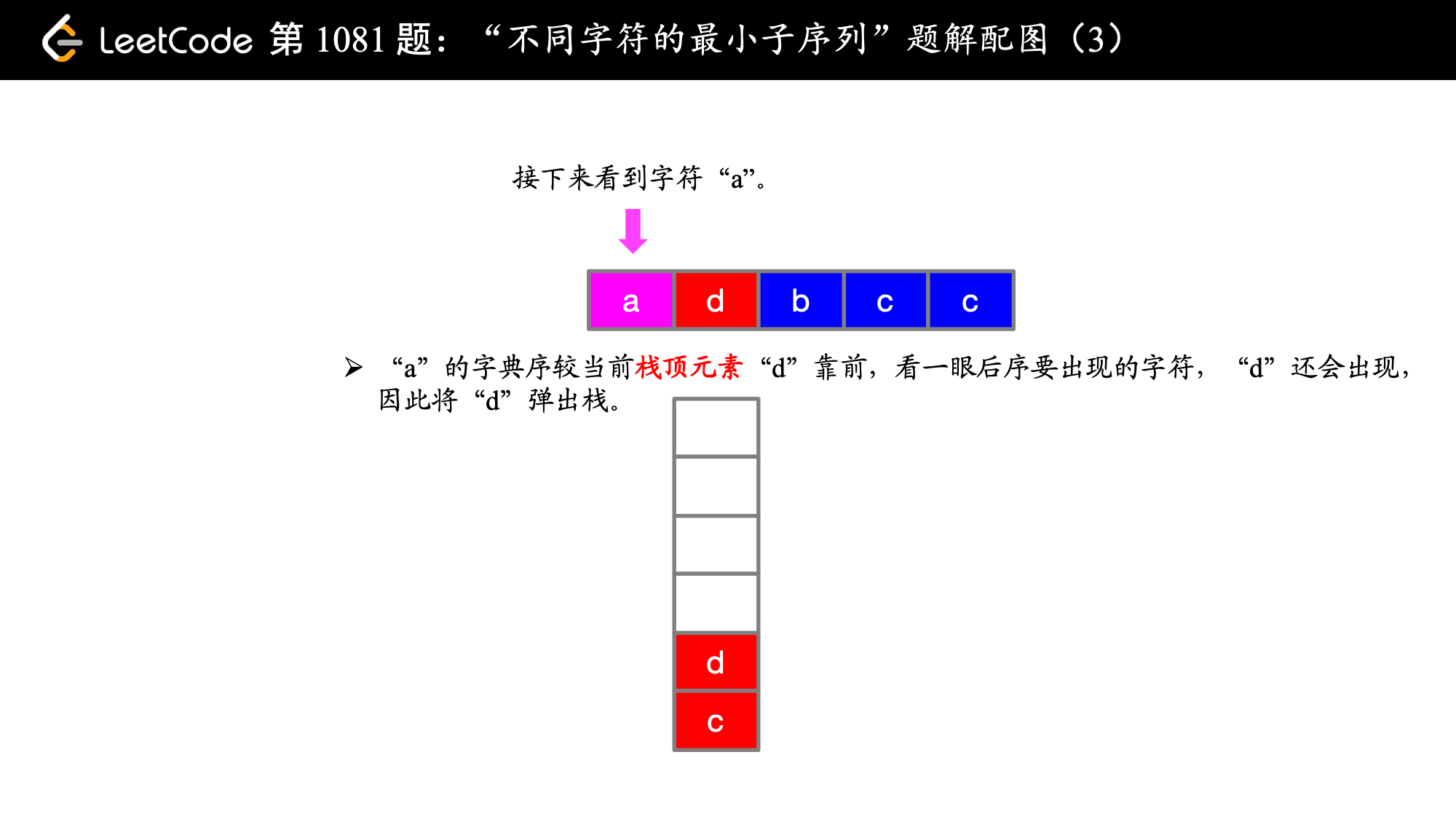),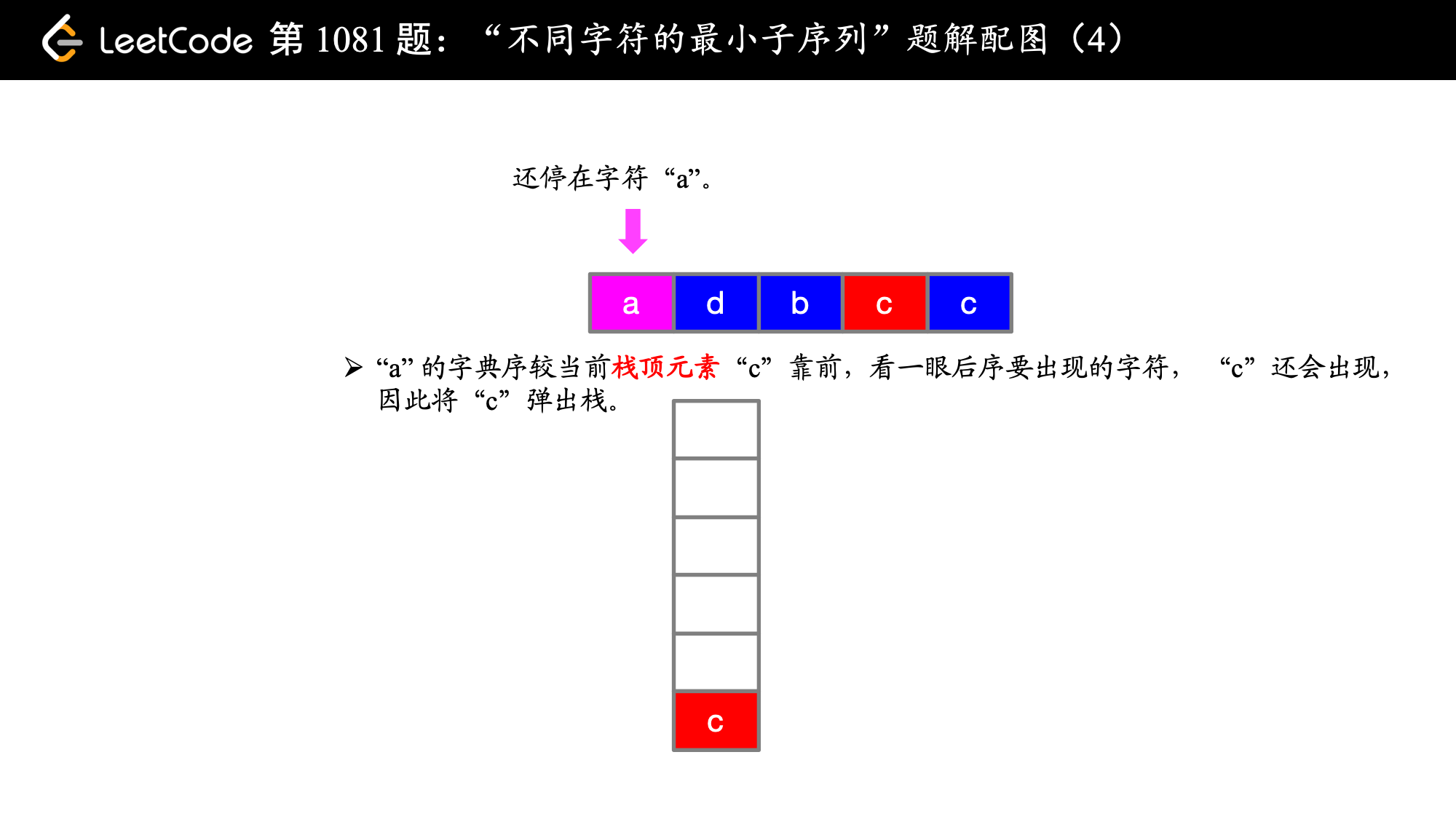),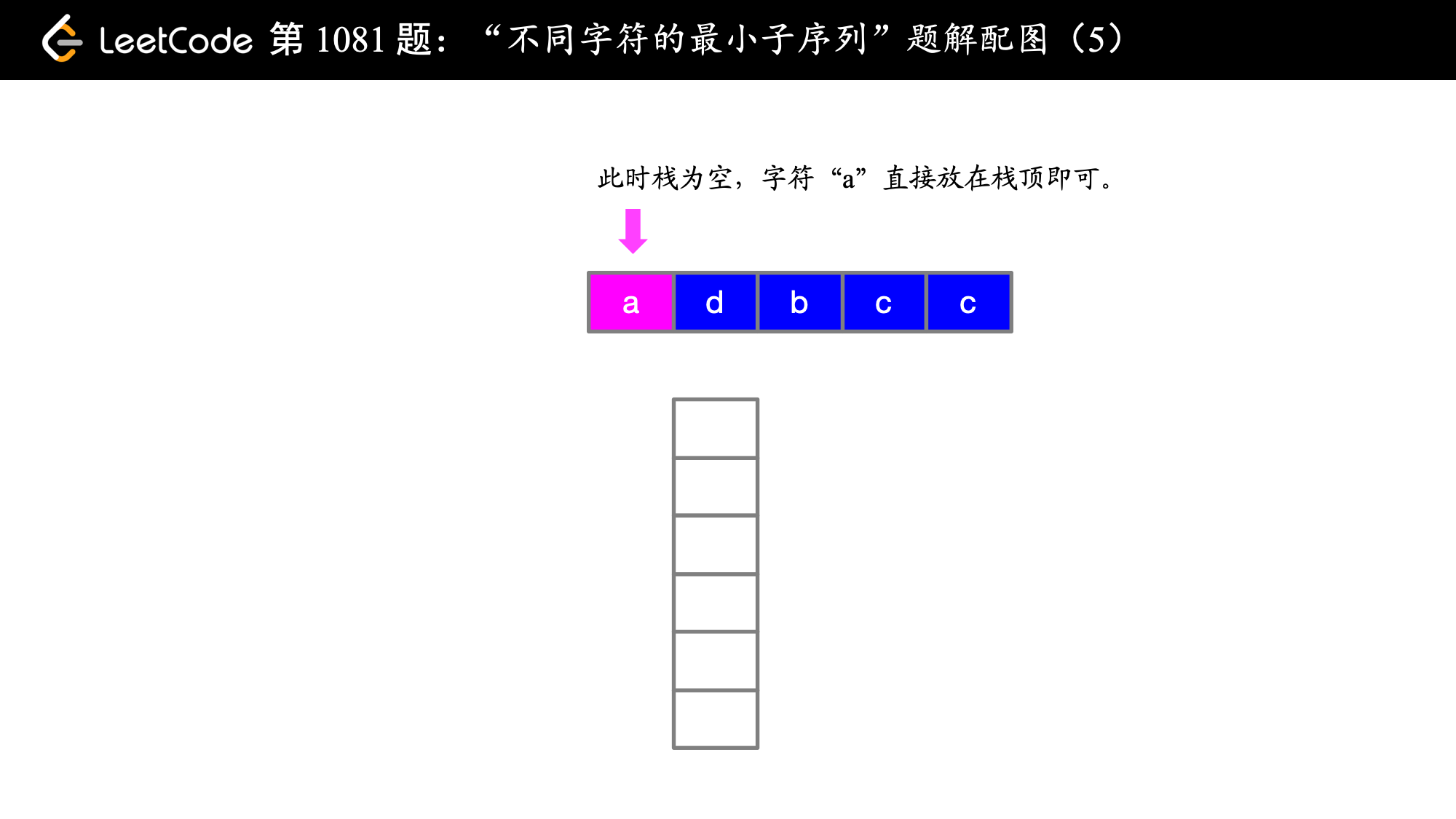),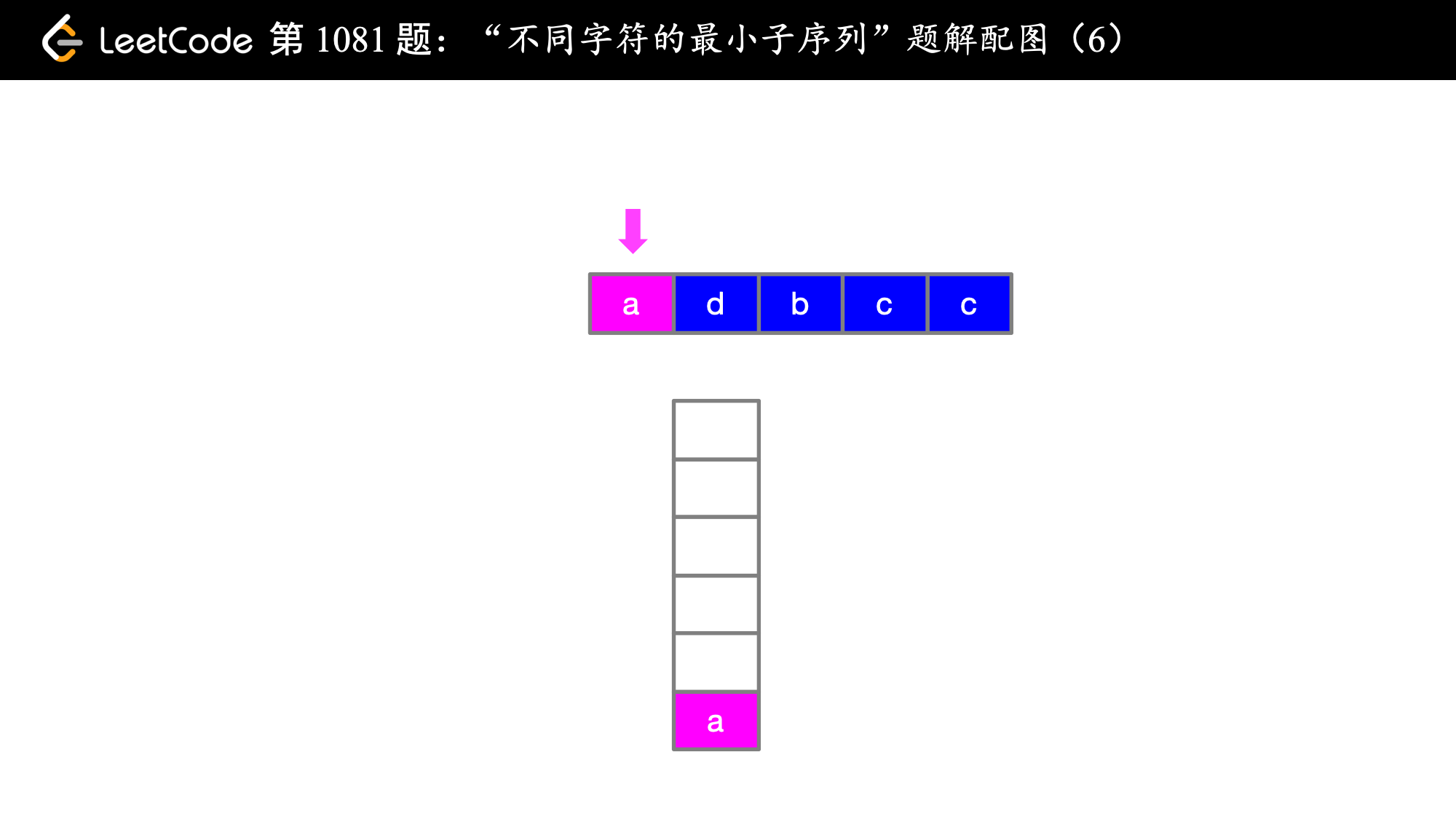),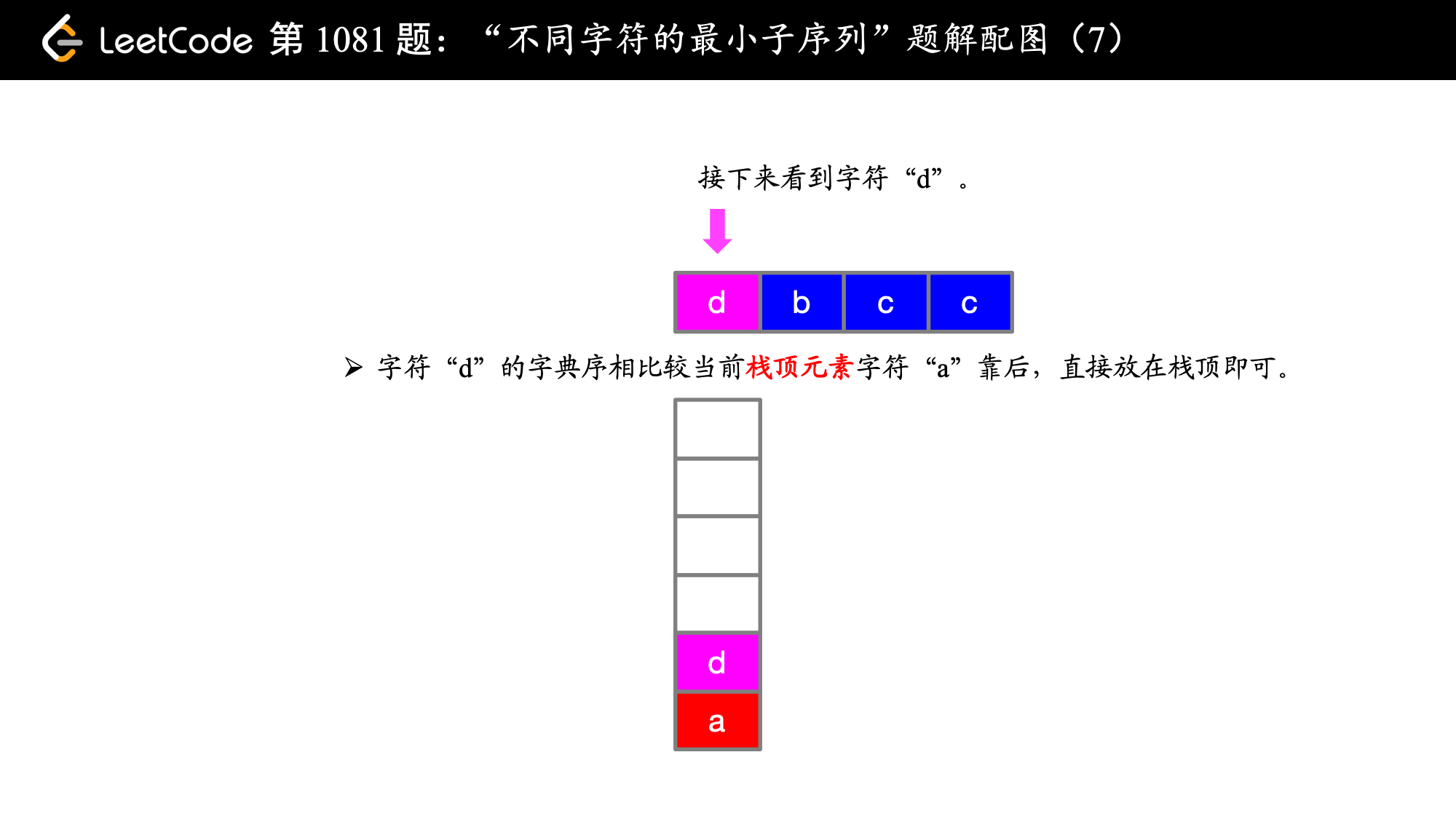),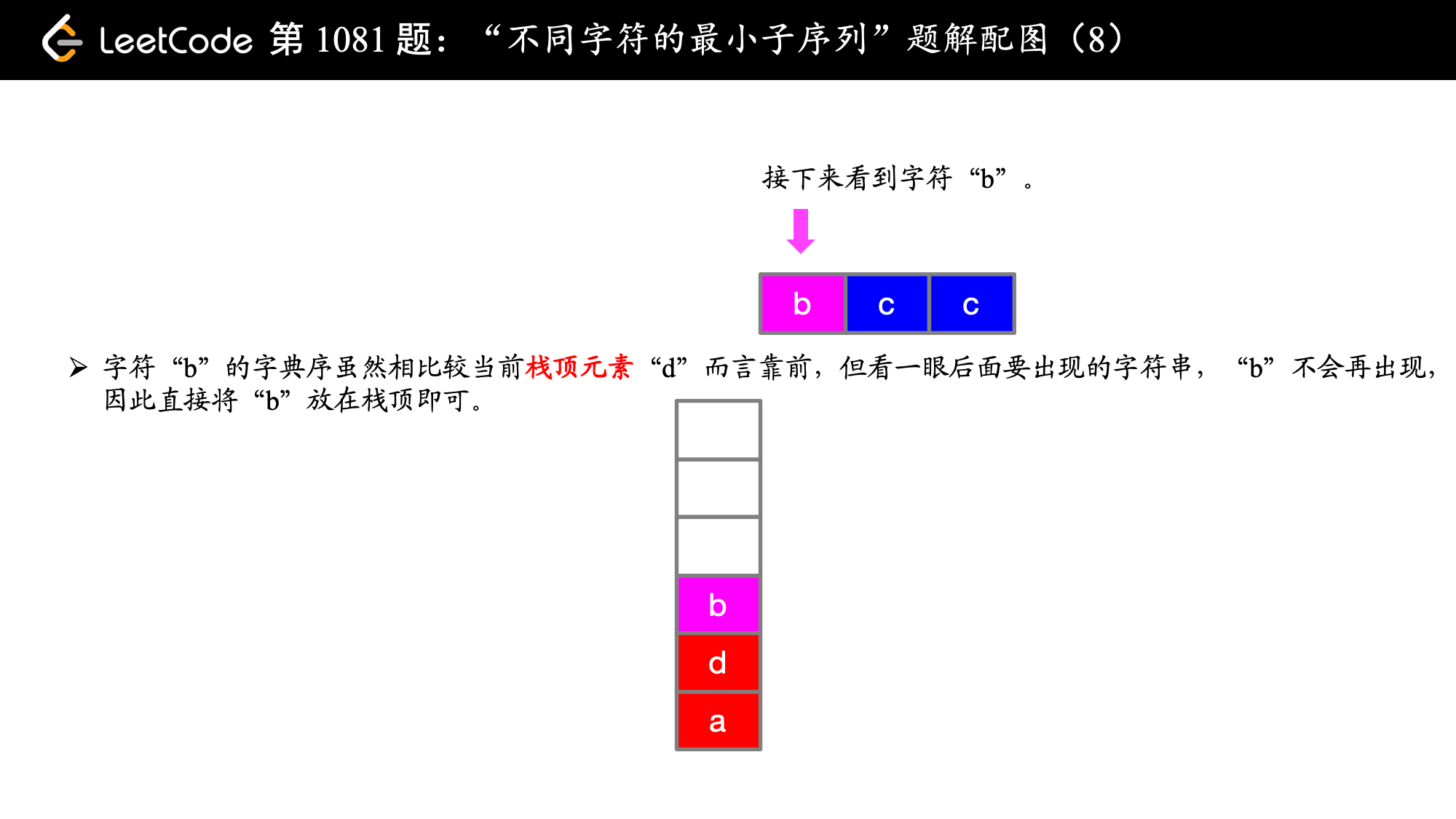),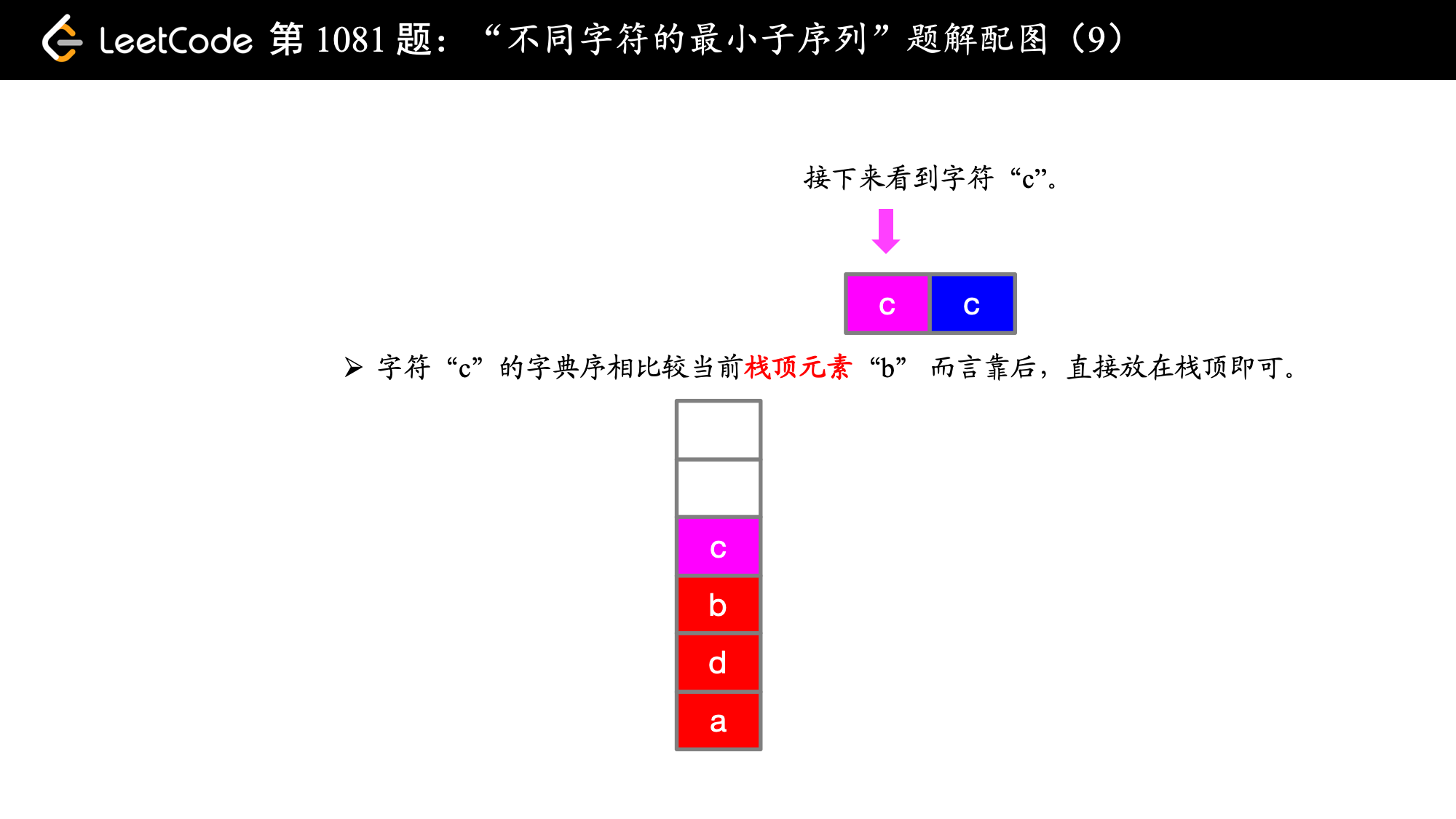),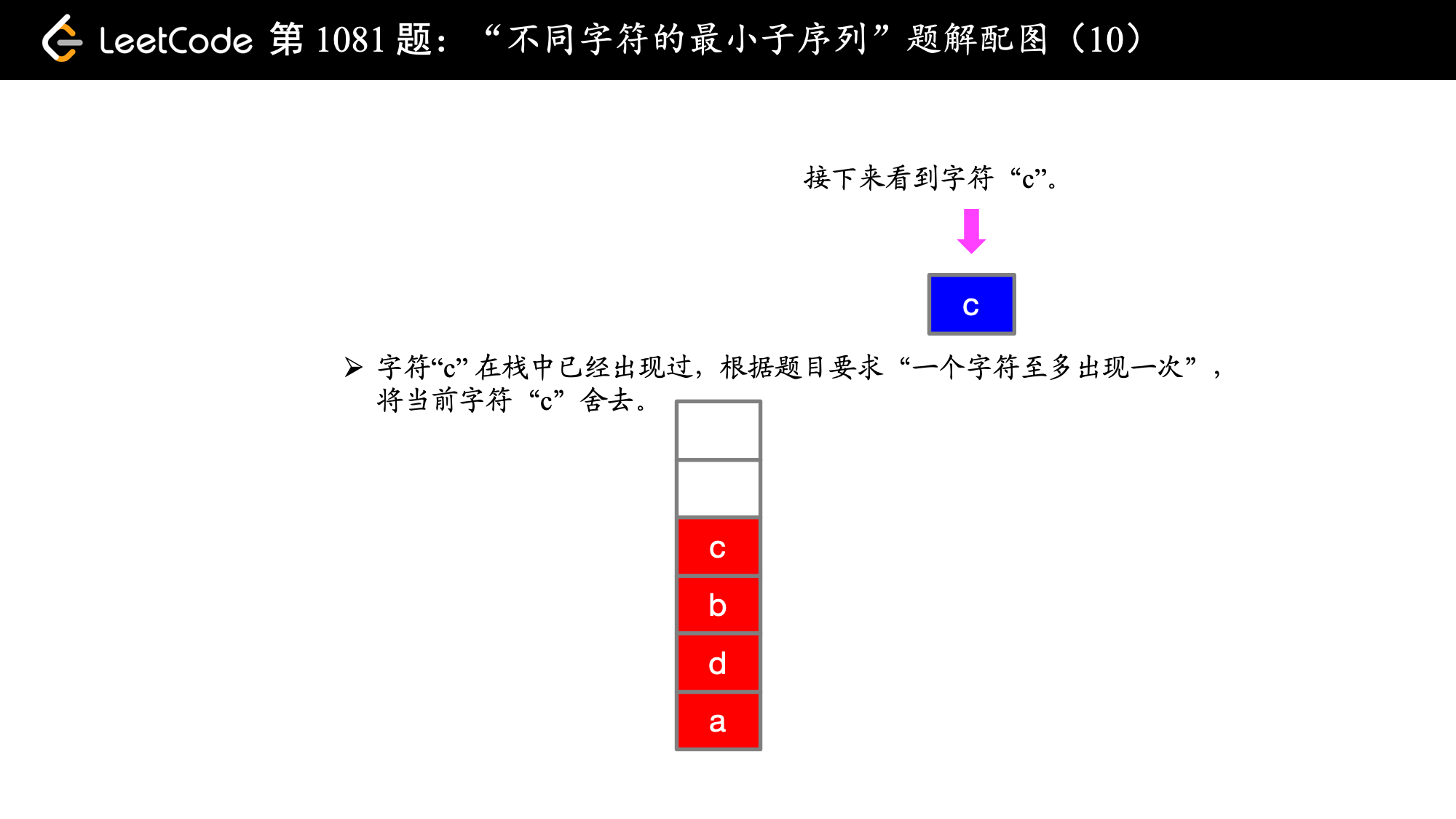### 编码实现

Python 代码：

class Solution:
def smallestSubsequence(self, text: str) -> str:
size = len(text)
stack = []
for i in range(size):
if text[i] in stack:
continue
while stack and ord(text[i]) < ord(stack[-1]) and \
text.find(stack[-1], i) != -1:
stack.pop()
stack.append(text[i])
return ''.join(stack)

Java 代码：

import java.util.Stack;

public class Solution {

public String smallestSubsequence(String text) {
int len = text.length();
Stack stack = new Stack<>();
for (int i = 0; i < len; i++) {
Character c = text.charAt(i);
if (stack.contains(c)) {
continue;
}
while (!stack.empty() && c < stack.peek() && text.indexOf(stack.peek(), i) != -1) {
stack.pop();
}
stack.push(c);
}
StringBuilder sb = new StringBuilder();
for (Character c : stack) {
sb.append(c);
}
return sb.toString();
}
}

Python 代码：

class Solution:
def smallestSubsequence(self, text: str) -> str:
size = len(text)
stack = []
pre = 0
# 从索引 i 到索引 size - 1 的位掩码
post =  * size

for i in range(size):
for j in range(i, size):
post[i] |= (1 << (ord(text[j]) - ord('a')))

for i in range(size):
# print(stack, text[i])
if pre & (1 << ord(text[i]) - ord('a')):
# print(i, pre & (1 << ord(text[i]) - ord('a')))
continue
while stack and ord(text[i]) < ord(stack[-1]) \
and post[i] & (1 << (ord(stack[-1]) - ord('a'))):
top = stack.pop()
pre ^= (1 << ord(top) - ord('a'))
pre |= (1 << ord(text[i]) - ord('a'))
stack.append(text[i])
return ''.join(stack)

Java 代码：

import java.util.Stack;

public class Solution {

public String smallestSubsequence(String text) {
int len = text.length();
// 从索引 i 到索引 size - 1 的位掩码
int[] post = new int[len];
int pre = 0;
Stack stack = new Stack<>();
for (int i = 0; i < len; i++) {
for (int j = i; j < len; j++) {
// System.out.println(text.charAt(j));
post[i] |= (1 << (text.charAt(j) - 'a'));
}
}

// System.out.println(Arrays.toString(post));
for (int i = 0; i < len; i++) {
Character c = text.charAt(i);
if ((pre & (1 << (c - 'a'))) > 0) {
continue;
}
while (!stack.empty() && c < stack.peek() &&
(post[i] & (1 << (stack.peek() - 'a'))) != 0) {
Character top = stack.pop();
pre ^= (1 << top - 'a');
}
pre |= (1 << c - 'a');
stack.push(c);
}
StringBuilder sb = new StringBuilder();
for (Character c : stack) {
sb.append(c);
}
return sb.toString();
}
}

上一篇「力扣」第 126 题：单词接龙 II
「力扣」第 126 题：单词接龙 II 链接：126. 单词接龙 II； 给定两个单词（beginWord 和 endWord）和一个字典 *wordList，找出所有从 *beginWord 到 endWord 的最短转换序列。转换需
2018-08-01「力扣」第 455 题：分发饼干
「力扣」第 455 题：分发饼干题解地址：贪心算法（两个方向） + 优先队列（Python 代码）。 说明：文本首发在力扣的题解版块，更新也会在第 1 时间在上面的网站中更新，这篇文章只是上面的文章的一个快照，您可以点击上面的链接看到其他网
2018-07-07
目录